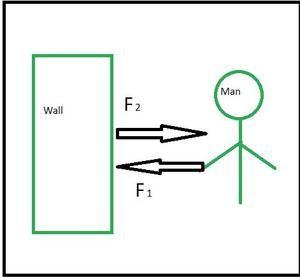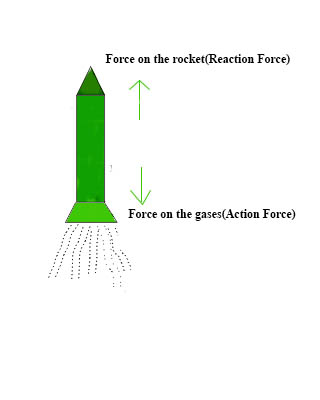# Newton’s Third Law of Motion

• Difficulty Level : Hard
• Last Updated : 22 Jun, 2021

It is important to understand that when you toss a ball against a wall, the ball exerts power on the wall. Similarly, the wall exerts a force on the ball, causing it to bounce off the wall. Similarly, the earth’s gravitational attraction pushes you down. What you may not understand is that you are also putting equal power on the ground. This amazing phenomenon is a result of Newton’s third law.

Newton’s third law states that if body A exerts a force on another body B, Body B must exert a force of equal size and opposite direction on body A. This law represents a specific symmetry in nature: forces always exist in pairs, and one body cannot exert a force on another without also experiencing a force. Let’s discuss it further.

### Newton’s Laws of Motion

In the 17th century, Sir Isaac Newton proposed the three laws of motion. These laws are commonly employed in classical mechanics. They are almost universally accepted. The laws are summarized here as:

• Newton’s First law states that a body at rest or uniform motion will continue to be at rest or uniform motion until and unless a net external force acts on it.
• Newton’s Second law states that the acceleration of an object as produced by a net force is directly proportional to the magnitude of the net force, in the same direction as the net force, and inversely proportional to the mass of the object.
• Newton’s third law states that for every action there is an equal and opposite reaction.

Here in the present article, only the third law of motion is properly explained. This law discusses the action-reaction pair of applied force.

### Newton’s Third Law of Motion

This law is stated as: “When one body exerts a force on another, the first body feels a force equivalent in the opposite direction of the force exerted.” According to the above statement, every interaction involves a pair of forces exerted on the interacting objects. The magnitudes of the forces are equal, and the force on the first item is directed in the opposite direction as the force on the second item.

Let’s understand this more clearly with the help of an example:

1. While moving on the ground, we push the ground backward by our feet. The ground also exerts a forward force on our feet of equal magnitude in the opposite direction which makes us move forward.
2. A book when lying on a table. The book exerts a downward force due to its weight, but it does not fall down, therefore the net force on the book is zero. This is because the table exerts an equal and opposite force on the book in the upward direction.

Explanation: Here in the above examples, we can see that two forces are acting on each of the bodies. In the first example, the force exerted by our feet on the ground is the action force and in response to that, the ground exerts an equal opposite force on our feet. In the second example, the force exerted by the weight of the book is the action force and the force exerted by the table on the book is the reaction force.

Now, let’s learn about two terms called Action and Reaction force, which are used in Newton’s third law of motion.

Action Force: The initial outside force exerted on the body is called the action force.

Reaction Force: The force the body exerts to respond to the action force in the opposite direction is called reaction force.Illustration of Newton’s third law of motion

From the above case Newton’s third law of motion can also be stated as:

If there is any interaction between two bodies (A and B), the force FAB (force applied by body B on body A) is equal to force FBA (force applied by body A on body A) but they are opposite in direction.

Note:

• The action and reaction force are exerted by different bodies and not on the same body.
• The action and reaction always occur simultaneously and they are always in pair.

### Examples of Action-Reaction Forces

Nature has a wide range of action-reaction pairings. Following are some examples listed below:

1. An example of an action-reaction pair is the propulsion of a fish through water. A fish’s fins are used to push water backward. This push serves to propel the fish ahead.
2. The magnitude of the force on the water equals the magnitude of the force on the fish; the magnitude of the force on the water (backward) is opposite to the magnitude of the force on the fish (forwards).
3. The bird’s flight is an example of an action-reaction pair. The air is pushed downward by the bird’s wings. The air is pushed higher by the air.
4. A swimmer pushes up against the water, and the water pushes back.
5. Helicopters generate lift by forcing the air downward, resulting in an upward reaction force.
6. Climbers use their vertical rope to propel themselves upwards.

### Real-life Examples of Application of Newton’s third law of motion

• The motion of a boat in the water: In water, the boatman pushes the water with his oar in the backward direction (action force) and in response to it, the water exerts an equal opposite force on the boat in the forward direction (reaction force). Thus the boat moves forward.
• Firing a bullet from a gun: When we fire a bullet from a gun, a force F is applied on the bullet(action force) and the gun experiences an equal recoil force (reaction force) in the opposite direction.
• Catching a ball: While catching a ball, the ball exerts a force on the hands of the player (action force) due to its weight and motion, the player also exerts an equal force on the ball to stop it in opposite direction.
• The motion of a rocket: Inside a rocket, fuels are burnt and the burnt gases at high temperature and pressure are expelled out, thus the rocket exerts a force on the gases to expel them out of the rocket through the nozzle(action force) of the rocket in a backward direction and the gases also exert a force on the rocket (reaction force) in the upward direction and thus helps the rocket in moving forward.### Sample Problems

Problem 1: A light ball falling on the ground after striking rises upwards, explain.

Solution:

A light ball when strikes the ground exerts force on the ground (action force) in the downward direction and thus, according to Newton’s third law of motion, the ground in response to it exerts an equal amount of force in opposite direction on the light ball (reaction force) and thus it rises upwards.

Problem 2: Name the action and reaction force in the following examples:

(a) A book lying on the table

(b) The motion of a rocket in an upward direction

(c) Firing a bullet from a gun

Solution:

The given examples are categorized as:

Problem 3: Explain the walking of a person on the ground with the help of Newton’s third law of motion.

Solution:

While walking on the ground, a person exerts a force on the ground in a backward direction with his feet (action force) and according to Newton’s third law of motion, the ground exerts an opposite and equal force in the forward direction as a reaction force, and so we can walk on the ground or on floor.

Problem 4: A man pushes a wall with a force of 100N towards the north. What force does the wall exert on the man?

Solution:

Given that,

The action force, F is 100 N.

According to Newton’s third law of motion,

Action force = – Reaction force

Therefore, the reaction force = -100 N

That is, the reaction force is equal to 100 N towards south.

Problem 5: A cricket ball of mass 500 g travelling at 20 m/s is hit by a cricket bat to return it along its original path with a velocity of 10 m/s. Calculate the change of momentum that occurred in the motion of the cricket ball by the force applied by the cricket bat.

Solution:

Given that,

The mass of the hockey ball, m is 500 g = 0.5 kg.

The initial velocity of the ball, u is 20 m/s.

The final velocity of the ball after hitting, v is 10 m/s.

The change in momentum = Final momentum – Initial momentum

= mv – mu

= m (v – u)

= 0.5 kg × (20 m/s – 10 m/s)

= 0.5 kg × 10 m/s

= 5 kg m/s

Therefore, change of momentum of the cricket ball by the force applied by the cricket bat is 5 kg m/s.

Problem 5: Name the principle that is obeyed by Newton’s third law of motion. Also, state the principle.

Solution:

Newton’s third law of motion obeys the principle of conservation of momentum. According to the principle of conservation of momentum, when no unbalanced external force acts on the system, the sum of initial momentum is exactly equal to the sum of the final momentum of the system.

My Personal Notes arrow_drop_up